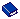APPLY DISCOUNT TO PACKAGES Application Setting

 Group.  Reservations Type.  Parameter Application.  PMS                      S&C                      OVOS

Description.  If this is set to Y, percentage discounts will be applied to packages. Amount discounts are not supported for packages. The Package discount is not applied when Post Rate Code option is used.

Property Determination.

 Activation / Inactivation Rules.

 Available Values. Y- Yes N- No

 Other Application Settings.

Affected Areas

 Menus / Screens / Fields. Billing>Options>Post Rate Code

 Reports & Report Forms.

 Permissions.

Other Areas.

Packages that are configured with the Add Rate Separate Line attribute will not be included in the discount calculation.

### Examples

Configuration

Rate: Discount

Rate Amount: \$100.00

Discount: Flagged

Package: Discount

Package Amount: \$20.00

###### Example 1

-Configured Discount package to be included in the rate.

-Total Rate Amount reflects as \$50.00

Total Rate \$100.00

\$80.00 room - 50% discount = \$40.00

\$20.00 package - 50% discount = \$10.00

Total Rate Amount = \$50.00

Discount calculated on room rate and package.

###### Example 2

-Configured Discount package to be added to the rate in a separate line.

-Total Rate Amount reflects as \$120.00

-Total Rate Amount reflects as \$70.00

Total Rate \$120.00

\$100.00 room - 50% discount = \$50.00

\$20.00 package - No discount applied = \$20.00

Total Rate Amount = \$70.00

No discount was calculated on the package.

###### Example 3

-Configured Discount package to be added to the rate in combined line-Added to reservation

-Total Rate Amount reflects as \$120.00

-Total Rate Amount reflects as \$60.00

Total Rate \$120.00

\$100.00 room - %50 discount = \$50.00

\$20.00 package - %50 discount = \$10.00

Total Rate Amount = \$60.00

Discount calculated on room rate and package.